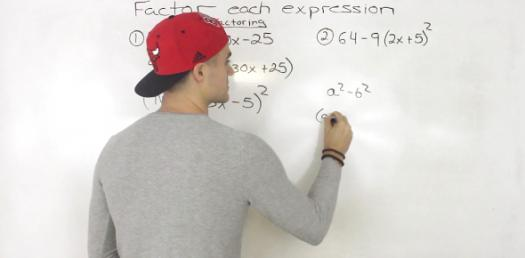20 Questions | Total Attempts: 664SettingsThe quiz below is based on a given algebraic equation. You are expected to try solving it and you will get a chance to solve the questions that follow that are the same. All the best as you do and if you fail keep trying until you get it right.

• 1.
N + 5 = 8
• A.

13

• B.

3

• C.

40

• D.

-3

• 2.
D - 7 = 1
• A.

8

• B.

-8

• C.

7

• D.

6

• 3.
2 = 5 - r
• A.

7

• B.

-3

• C.

3

• D.

-1

• 4.
13 = 3 + c
• A.

10

• B.

16

• C.

-10

• D.

-16

• 5.
P - 4 = 3
• A.

12

• B.

1

• C.

7

• D.

-1

• 6.
9 = r - 3
• A.

27

• B.

3

• C.

6

• D.

12

• 7.
3n = 15
• A.

12

• B.

5

• C.

45

• D.

18

• 8.
11r = 11
• A.

11

• B.

0

• C.

1

• D.

22

• 9.
55 = 11m
• A.

5

• B.

44

• C.

66

• D.

605

• 10.

• A.

10

• B.

4

• C.

21

• D.

11

• 11.
• A.

8

• B.

27

• C.

21

• D.

11

• 12.
What should be done to solve the following equation? m + 3 = 25
• A.

Subtract 3 from both sides

• B.

• C.

• D.

Multiply 25 x 3

• 13.
What should be done to solve the following equation?
• A.

• B.

Subtract 4 from both sides

• C.

Multiply 4 x 10

• D.

Divide 10 by 4

• 14.
What should be done to solve the following equation? a - 20 = 5
• A.

• B.

Divide both sides by 20

• C.

Multiply both sides by 20

• D.

Subtract 20 from both sides

• 15.
What should be done to solve the following equation?
• A.

Multiply d x 7

• B.

Subtract 63 - 7

• C.

• D.

Divide 63 by 7

• 16.
7y means ___________
• 17.
To solve equations, use ________________ operations.
• 18.
When solving equations, whatever is done to one side of the equation must be done to the ___________________________.
• 19.
To solve this equation,  , you would ____________
• 20.
To solve this equation, , you would __________
Related TopicsBack to top